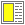# Exercise solution: Construction of symbolic expressions

The following is a name binding form with the left and right branch of the structure:

```(let ((left (cons (cons 'a 'b) (cons 'c 'd)))
(right (cons (cons 'e 'f) (cons 'g 'h))))
(cons left right))```

It is, of course, also possible to make one nasty expression with cons'es:

`(cons (cons (cons 'a 'b) (cons 'c 'd)) (cons (cons 'e 'f) (cons 'g 'h)))`

Please notice the way the Scheme interpreter prints the value of the expression. Try find the rule it applies for printing.